## The Discrete Charm of the Fourier Transform

2015 September 30

The other day, I picked up the latest copy of the CAS’ journal, Variance and skipped to the back where Leigh Halliwell had an article. I hope that I’m well on record as being one of his biggest fans, but if not, let me remedy that now. Leigh Halliwell has done really tremendous stuff. He’s mathematically sophisticated, but addresses practical problems. I often struggle to keep up with his flurry of ideas and deft handling of the math, but once I (possibly) sort it out, it becomes embedded in my thinking.

This most recent piece is fairly short and only addresses the basic mechanics of the discrete Fourier transform. I’ve used the Fourier transform before (even helped write a paper which uses it), but I’ve not touched it in ages. My understanding is rusty. Further, I’ve done nothing at all with complex numbers in R, so this will be interesting.

My first goal is to visualize the second section of Halliwell’s paper. This is nothing more than describing the idea of “roots of unity”. A couple moments with Google informs me how to construct a complex number in R. With that, I can knock together a short function to generate roots. Let’s have a look:

``````RootsOfUnity <- function(n){
k <- seq(0, n-1)
exponent <- complex(imaginary = 2 * pi * k / n)
w <- exp(exponent)
w
}

Roots2 <- function(n){
k <- seq(0, n-1)
theta <- 2 * pi * k / n
w <- complex(real = cos(theta)
, imaginary = sin(theta))
w
}``````

There’s no real reason to create two functions. By Euler’s formula they’re identical. I was curious if there were any machine precision issues that might favor one approach. According to `R`, the results are identical.

``````myRoots <- lapply(1:10, RootsOfUnity)
myRoots2 <- lapply(1:10, Roots2)

identical(myRoots, myRoots2)``````
``##  TRUE``
``````myProducts <- sapply(myRoots, prod)
mySums <- sapply(myRoots, sum)

myProducts``````
``##    1+0i -1+0i  1-0i -1+0i  1-0i -1+0i  1-0i -1+0i  1-0i -1+0i``
``mySums``
``````##    1.000000e+00+0.000000e+00i  0.000000e+00+1.224647e-16i
##   -2.220446e-16+3.330669e-16i -1.224396e-16+1.225148e-16i
##   -2.220446e-16+1.110223e-16i  0.000000e+00+4.555818e-16i
##   -1.387779e-16+1.110223e-16i -3.445052e-16+1.149254e-17i
##   -7.771561e-16+1.110223e-16i -3.330669e-16+1.225148e-16i``````

Note that after n=1, the sums don’t quite agree with Halliwell’s math. Of course the smart money is on Halliwell (and I certainly can’t fault his algebra). This may be an issue of precision.

Sets of numbers are fine, but all those plusses and minuses and letter “i”s are freaking me out. Let’s look at these numbers visually. To reinforce the intuition, we’ll draw a unit circle and confirm that the points do indeed lie there. I’ll construct a list of data frames to hold the values as `ggplot2` tends to be happier with them. I also did a few experiments (not shown) plotting the modulus and argument values with polar coordinates.

``````library(ggplot2)
lstDF <- lapply(myRoots, function(x){
data.frame(Mod = Mod(x), Arg = Arg(x), Real = Re(x), Imaginary = Im(x))
})

PlotRoots <- function(df, aCircle){
plt <- ggplot(df, aes(x = Real, y = Imaginary)) + geom_point(color = "red")
plt <- plt + geom_path(data = aCircle, aes(x = x, y = y))
plt
}

t <- seq(0, 2*pi, length.out = 200)
myCircle <- data.frame(x = cos(t), y = sin(t))

plt <- PlotRoots(lstDF[], myCircle)
plt``````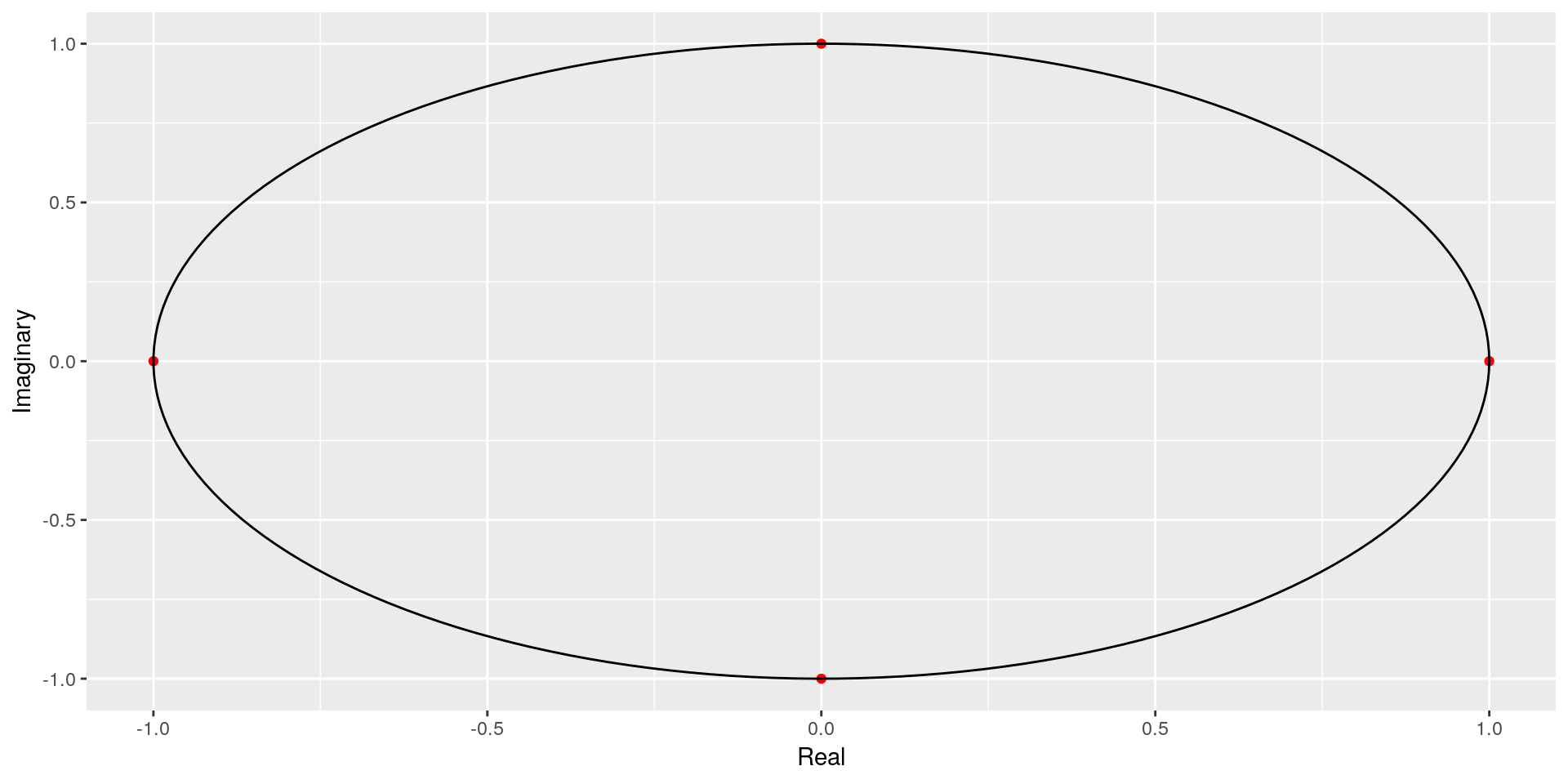``````plt <- PlotRoots(lstDF[], myCircle)
plt``````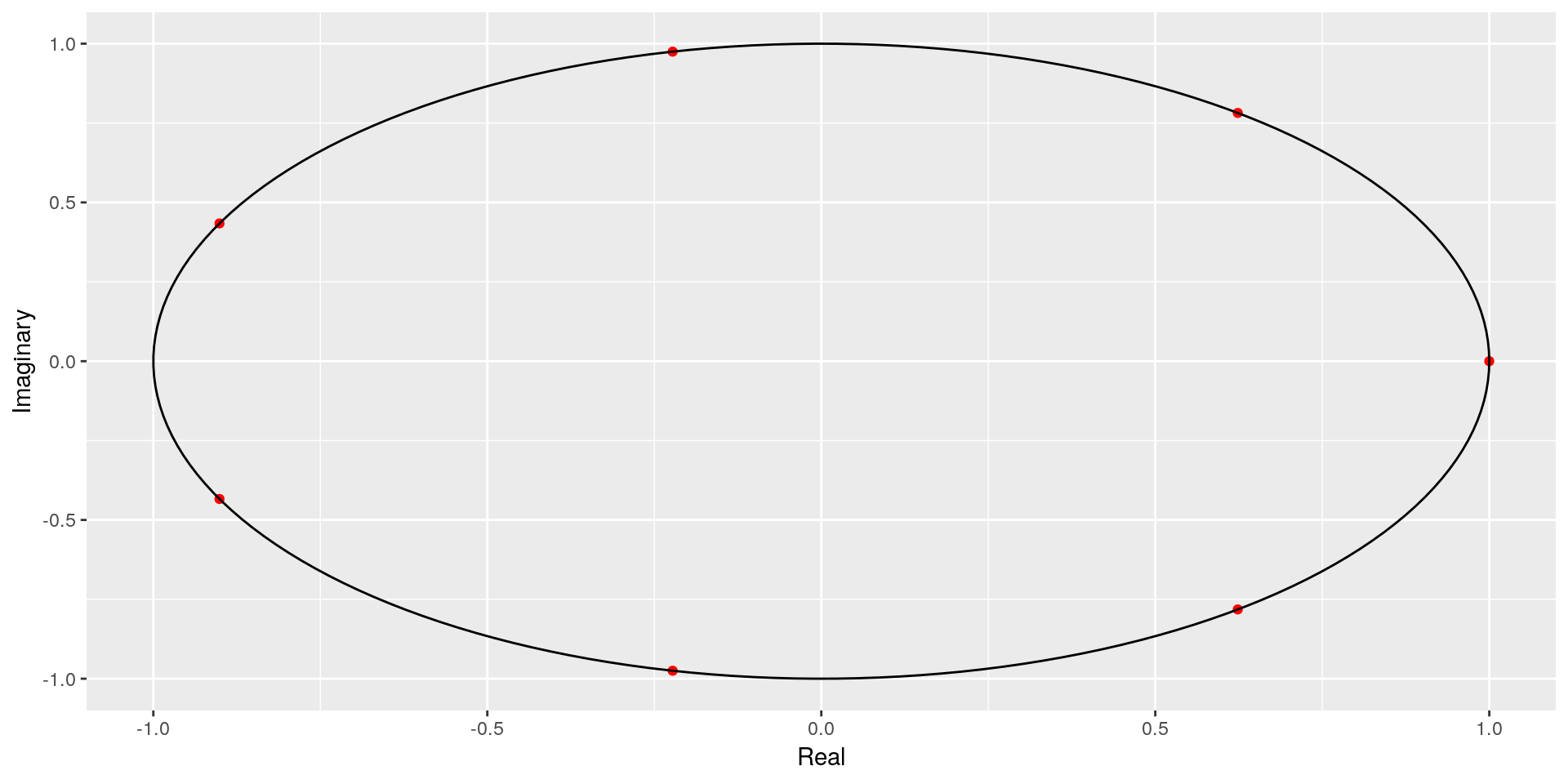So, here we have a visual representation of Halliwell’s main point: the Fourier transform is periodic for fixed `n`. If you need more points, we’ll just keep going around the unit circle to get them. This is something we can grasp intuitively by referring to Euler’s formula, which is the sum of sine and cosine functions, which are periodic. Let’s draw one last plot where we show 8 points and also 4096.

``````myRoots <- lapply(c(8, 4096), RootsOfUnity)

df1 <- data.frame(Real = Re(myRoots[]), Imaginary = Im(myRoots[]))
df1\$Set <- 8

df2 <- data.frame(Real = Re(myRoots[]), Imaginary = Im(myRoots[]))
df2\$Set <- 4096

df <- rbind(df1, df2)
df\$Alpha <- 1/ df\$Set

plt <- ggplot(df, aes(x = Real, y = Imaginary, color=Set, alpha = 1/Set)) + geom_point()
plt + theme(legend.position = "none")``````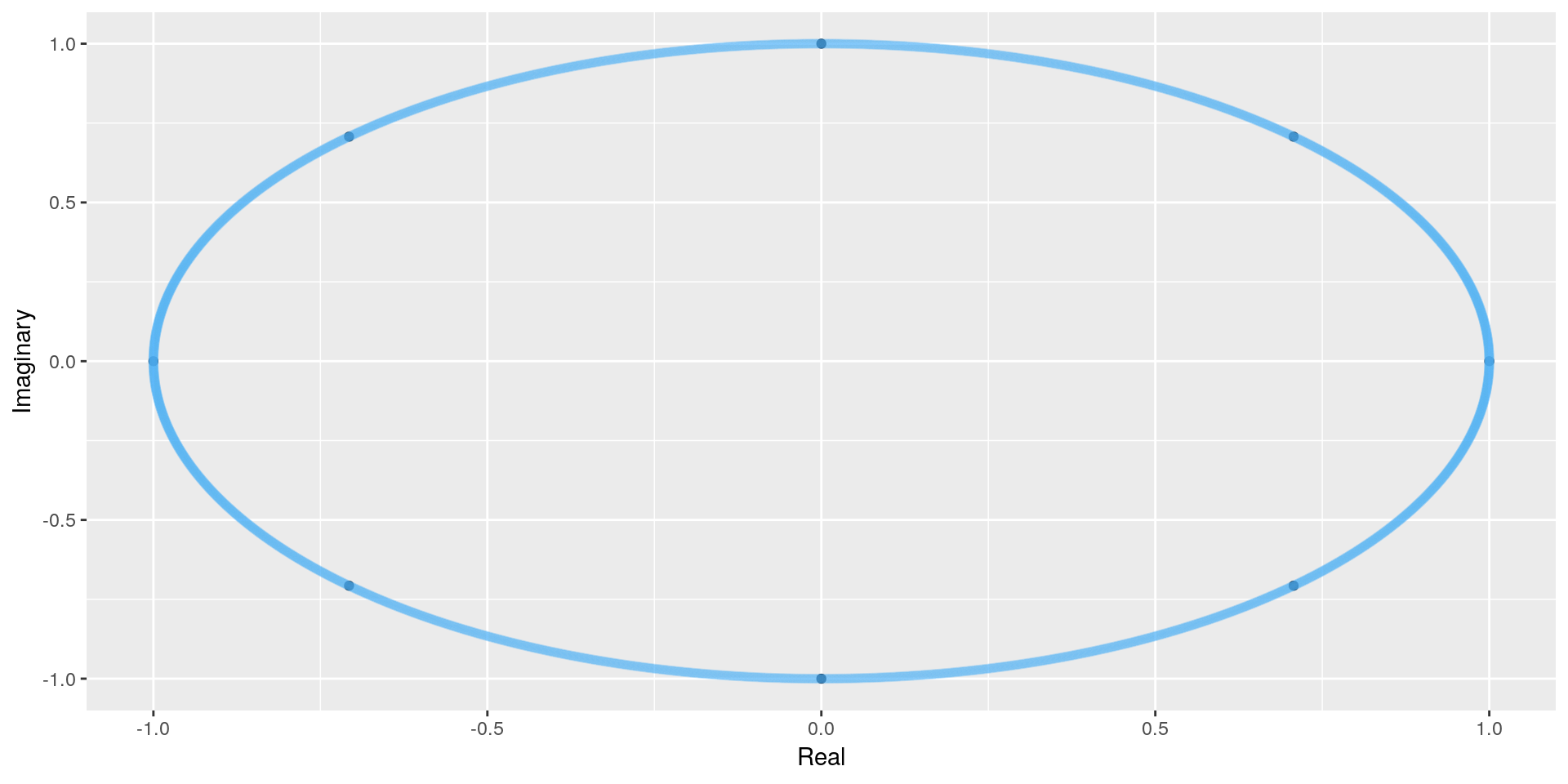By fiddling with the transparency, we can see the smaller set overlaid on the larger one. If we’re only using 8 points, we’ll go round that circle pretty quickly. With 4096, we won’t.

OK, so circles are fun, but the real important bit is how it can help us price insurance. It’s been ages since I translated Heckman and Meyer’s paper from Fortran to VBA and my brain is a bit foggy. For a warmup, we’ll reproduce Halliwell’s example of convolving a binomial process. We’ll use the same construction he applies; namely a vector with four values and we’ll push it farther than it ought to go so that we can observe the cyclicality.

``````px <- c(0.5, 0.5, 0, 0)
powers <- 2:5
pc <- lapply(powers, function(x){
x <- fft(fft(px)^x, inverse=TRUE) / length(px)
x <- Re(x)
})

pc <- matrix(unlist(pc), nrow=4)``````
 0.25 0.125 0.125 0.188 0.5 0.375 0.25 0.188 0.25 0.375 0.375 0.312 0 0.125 0.25 0.312

So, we got exactly what Halliwell got. It’s wrong, of course, so let’s pad things a bit and get proper probabilities. In this case, we know that we’ll tap out at 6 elements, but let’s go ahead and pad to 8.

``````px <- c(px, rep(0, 2))
pc <- lapply(powers, function(x){
x <- fft(fft(px)^x, inverse=TRUE) / length(px)
x <- Re(x)
})

pc <- matrix(unlist(pc), nrow=6)``````
 0.25 0.125 0.0625 0.0312 0.5 0.375 0.25 0.156 0.25 0.375 0.375 0.312 3.2e-17 0.125 0.25 0.312 0 0 0.0625 0.156 -3.2e-17 -2.78e-17 -1.85e-17 0.0312

I’m warmed up and ready to hit his last example: an aggregate set of losses where the number of claims is Poisson distributed, lambda = 3 and the severity is a very simple set of four values (the first of which corresponds to a claim value of zero).

``````PoissonPGF <- function(x, lambda){
x <- lambda * (x - 1)
x <- exp(x)
x
}

# In running the inverse, note that I have to divide the results by n.
# Halliwell points out this distinction in his seventh footnote.
FormS <- function(px, n){
px <- c(px, rep(0, n-4))
px <- fft(px)
px <- PoissonPGF(px, 3)
s <- Re(fft(px, inverse=TRUE) / n)
}

px <- c(0, 0.5, 0.4, 0.1)

aggs <- lapply(c(8, 4096), FormS, px = px)

library(dplyr)
df <- data.frame(Set = c(rep(8, 8), rep(4096, 4096)), x = c(1:8, 1:4096), y = unlist(aggs))
plt <- ggplot(data=filter(df, x<=30), aes(x=x, y=y, color=as.factor(Set))) + geom_point()
plt``````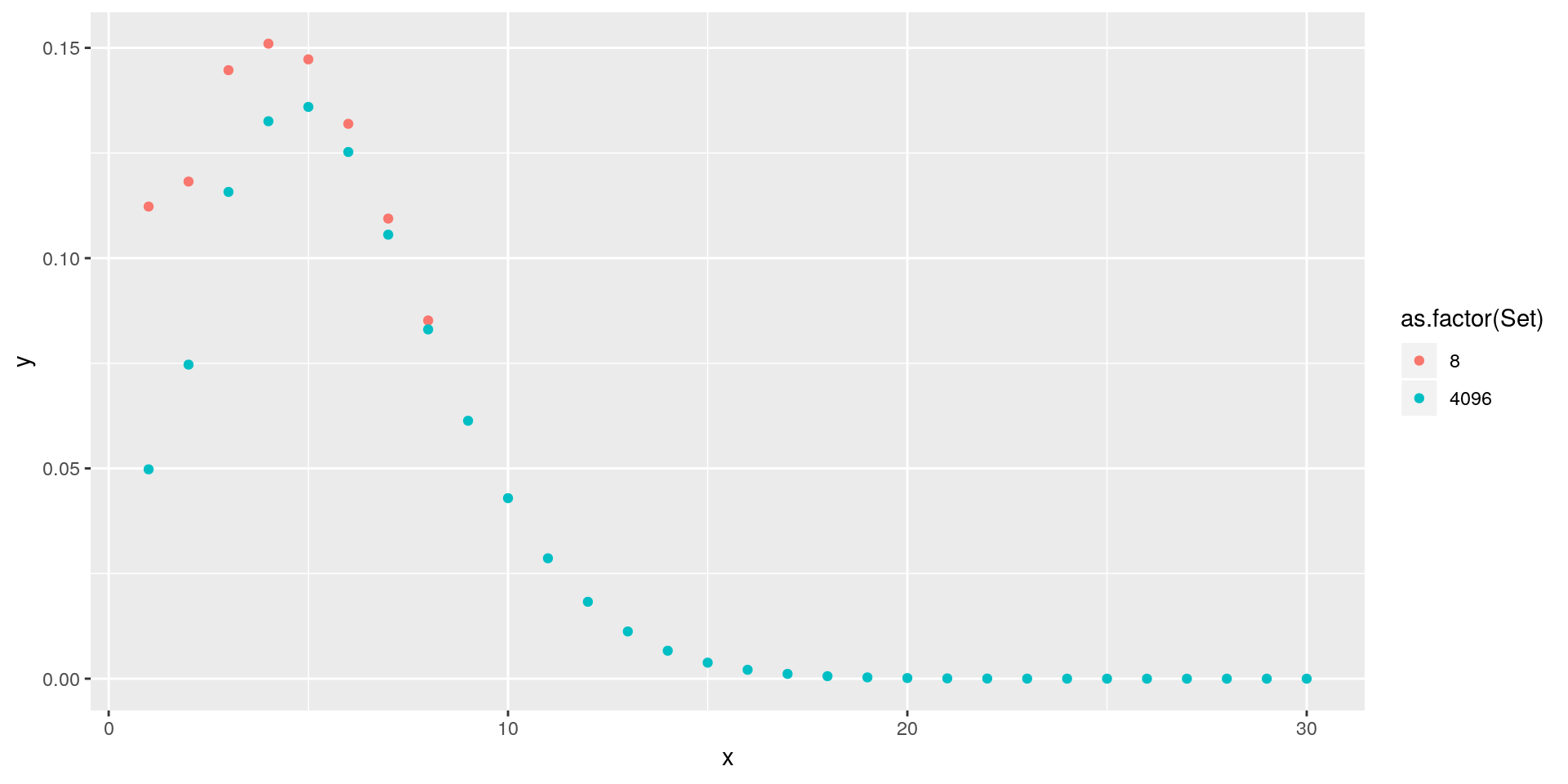Here we see a very clear graphic presentation of Halliwell’s point on cyclical overflow. The difference is greatest at the leftmost point of the graph. Points 7 and 8 almost agree. We’ll also note that 4096 is (for this range of values) probably overkill. The probability drops off substantially after x=30. Just for fun, let’s compare n=32 to n=4096

``````aggs <- lapply(c(32, 4096), FormS, px = px)

df <- data.frame(Set = c(rep(32, 32), rep(4096, 4096)), x = c(1:32, 1:4096), y = unlist(aggs))
df\$Set <- as.factor(df\$Set)
plt <- ggplot(data=filter(df, x<=32), aes(x=x, y=y, color=Set, shape=Set)) + geom_point()
plt``````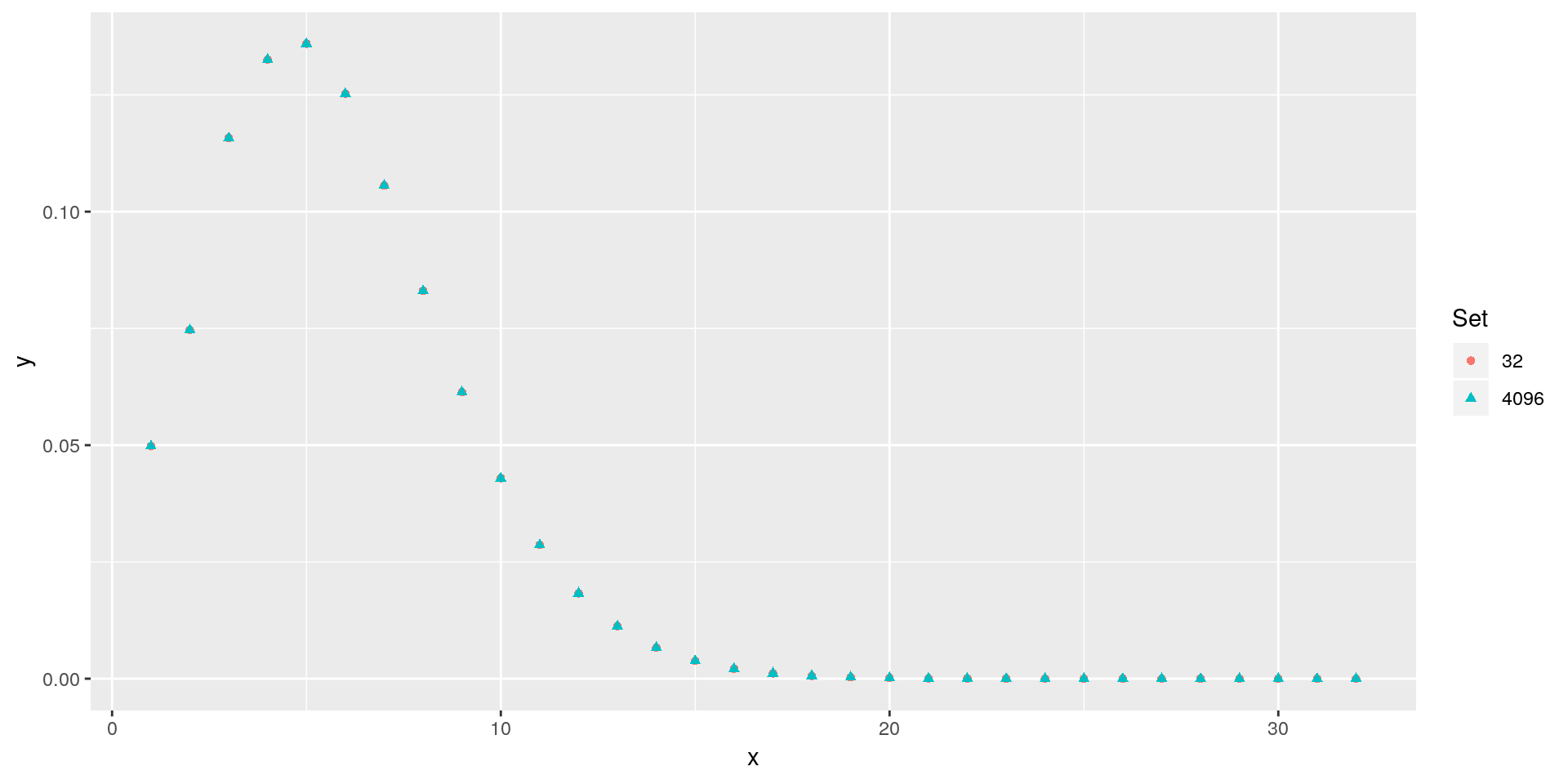``````library(tidyr)
df <- filter(df, x<=32) %>%
spread(Set, y)``````

Let’s compare the first 15 rows.

x 32 4096
1 0.0498 0.0498
2 0.0747 0.0747
3 0.116 0.116
4 0.133 0.133
5 0.136 0.136
6 0.125 0.125
7 0.106 0.106
8 0.0831 0.0831
9 0.0613 0.0613
10 0.0429 0.0429
11 0.0286 0.0286
12 0.0183 0.0183
13 0.0112 0.0112
14 0.00666 0.00666
15 0.00381 0.00381

One final thing. At one point, Halliwell asks us to imagine that we’re dealing with an infinite sum. This is impossible as there can’t be infinite roots of unity, so we have to cut things off somewhere. But his point is significant. No matter how many points we’re assuming, it is really an infinite set; a circle has no end.

Let’s have another look at our set of 8 points.

``````aggs <- lapply(c(8, 4096), FormS, px = px)
bigVector <- aggs[]
littleVector <- matrix(bigVector, nrow=8) %>%
rowSums()

dfCompare <- data.frame(Little = aggs[], Big = littleVector)``````
Little Big
0.112 0.112
0.118 0.118
0.145 0.145
0.151 0.151
0.147 0.147
0.132 0.132
0.109 0.109
0.0852 0.0852

This was loads of fun! I’ve gotten reacquainted with a technique I haven’t used in ages and I can’t wait to turn it loose on some actual data. `R` is flexible enough that switching between severity and frequency distributions is a breeze and the visualization makes it very easy to get a handle on how to tune results. So much fun!